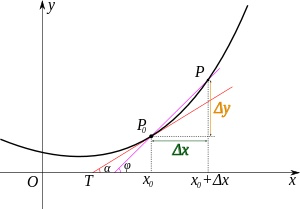# 看完这篇让你高数不挂科之——泰勒公式

2019/04/10 10:10

<br>

<br>

<br>

<br>

## 泰勒公式的定义

<br>$P_n(x)=a_0+a_1(x-x_0)+a_2(x-x_0)^2+\cdots+a_n(x-x_0)^n$

\begin{aligned} P_n(x_0) &= f(x_0), P_n'(x_0) = f'(x_0) \ P_n''(x_0) &= f''(x_0), \cdots, P_n^{(n)} = f^{(n)}(x_0) \end{aligned}

\begin{aligned} a_0 = f(x_0), 1\cdot a_1 = f'(x_0) \ 2!\cdot a_2 = f''(x_0), \cdots, n!\cdot a_n = f^n(x_0) \end{aligned}

$P_n(x)=f(x_0)+f'(x_0)(x - x_0)+\frac{f''(x_0)}{2!}(x - x_0)^2+\cdots+\frac{f^{(n)}}{n!}(x-x_0)^n$

<br>

## 泰勒公式的证明

<br>

$\frac{f(b) - f(a)}{F(b) - F(a)}=\frac{f'(\xi)}{F'(\xi)}$

$\frac{R_n(x)}{(x-x_0)^{n+1}}=\frac{R_n(x)-R_n(x_0)}{(x-x_0)^{n+1}-0}=\frac{R'_n(\xi_1)}{(n+1)(\xi_1-x_0)^n}, (\xi_1 \in (x_0, x))$

$\frac{R_n'(\xi_1)}{(\xi_1 - x_0)^n}=\frac{R'_n(\xi_1)-R_n'(x_0)}{(n+1)(\xi_1-x_0)^n-0}=\frac{R''_n(\xi_2)}{n(n+1)(\xi_2-x_0)^{n-1}}, (\xi_2 \in (x_0, \xi_1))$

$\frac{R_n(x)}{(x-x_0)^{n+1}}=\frac{R^{(n+1)}_n(\xi)}{(n+1)!}, (\xi \in (x_0, \xi_n))$

$R_n(x)=\frac{f^{(n+1)}(\xi)}{(n+1)!}(x-x_0)^{n+1}, (\xi \in (x_0, x))$

<br>

## 证明一下误差

<br>

$\lim_{x\to x_0}\frac{R_n(x)}{(x-x_0)^n} \leq \lim_{x\to x_0} \frac{\frac{M(x-x_0)^{n+1}}{(n+1)!}}{(x-x_0)^n}=\lim_{x\to x_0}\frac{M(x-x_0)}{(n+1)!}$

$f(x)=f(x_0)+f'(x_0)(x - x_0)+\frac{f''(x_0)}{2!}(x - x_0)^2+\cdots+\frac{f^{(n)}}{n!}(x-x_0)^n+R_n(x)$

$f(x)=f(0)+f'(0)x + \frac{f''(0)}{2!}x^2+\cdots + \frac{f^{(n)}(0)}{n!}x^n+\frac{f^{(n+1)}(\theta x)}{(n+1)!}x^{n+1}, (0 < \theta < 1)$

<br>

## 举例

<br>

$f'(0)=f''(0)=f'''(0)=\cdots =f^{(n)}(0)=1$

$e^x=1 + x + \frac{1}{2!}x^2+\frac{1}{3!}x^3+\cdots+\frac{1}{n!}x^n+\frac{e^{\theta x}}{(n+1)!}x^{n+1}$

$e^x\approx 1 + x + \frac{1}{2!}x^2+\frac{1}{3!}x^3+\cdots+\frac{1}{n!}x^n$

$\frac{e^{\theta x}}{(n+1)!}x^{n+1}\leq \frac{e}{11!}<\frac{3}{11!}$

<br>

## 思考

<br>### 作者的其它热门文章

0
0 收藏

0 评论
0 收藏
0